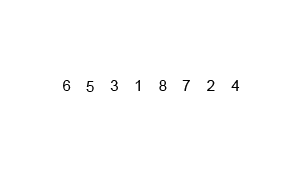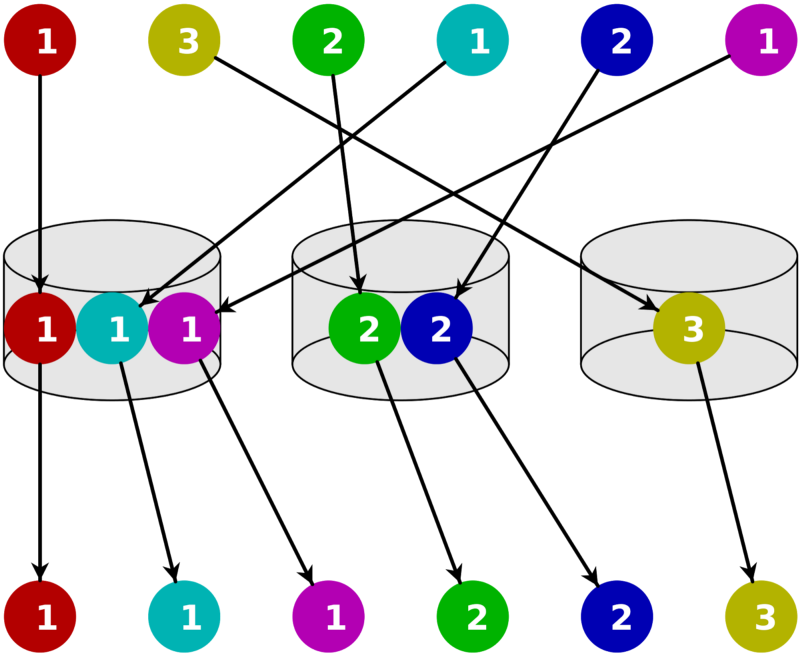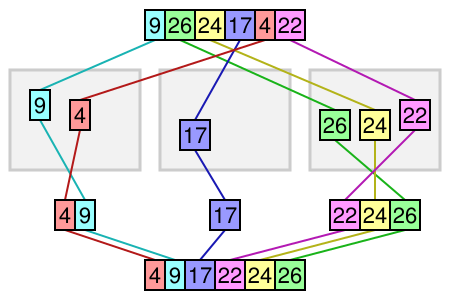Sorting in linear time -- the demise of Mergesort?

What is the fastest way to sort a deck of cards?

While hanging out at RC last week, Byung asked me a question: "What is the fastest way to sort a deck of cards? I thought this might be a trick question, because you aren't going to beat the beauty, simplicity, and speed of Mergesort, which sorts an array of n items in Θ(n log n) with a beautifully simple divide-and-conquer method. And that's that -- you can't sort a randomly unsorted array any faster than n log n.Byung smiled at me and replied: Actually, you can sort a deck of cards in Θ(n), using Bucket Sort.

No Byung, that's not how the world works. Θ(n log n) is the fastest sorting method. But tell me more.

The fastest way to sort a deck of cards is to first lay out a sorted array of buckets where each card will go. Then, you iterate straight through the cards, and put each card in it's respective bucket. Θ(n).No. Wow. It's makes so much sense. It's terribly efficient. But how? How did I not know what Bucket Sort was? Why is Mergesort taught at all? Just because it's so beautiful? Isn't mergesort what most programming languages use when you `.sort()`? Answer: kinda -- python uses timsort, which is a hybrid of Merge and Insertion sort. Did the core python devs make a mistake? Is culture holding back efficient programming? Or was the super simple Bucket Sort just discovered? Did Byung discover it? Are computers about to get far faster? log n faster? Did an AI discover it? So that it can become sentient log n faster? Is Bucket Sort going to save or destroy us?

Before my thoughts could spiral any further out of control, Byung consoled me: it's because we have extra information about the deck of cards... we know the range.

The range can be found in Θ(n), so that doesn't seem to be the issue. But I quickly came crashing back down to earth... there must be tradeoffs. So we started poking holes in how great Bucket Sort really is. Maybe it's not that good -- it can't be, right?

Let's start by organizing an array of numbers.

### Incredibly dumb version:

``````const bucketSort = (nums, range) => {
const low = range ? range : Math.min(...nums); // Θ(n) if needed
const high = range ? range : Math.max(...nums); // Θ(n) if needed
const buckets = []
for (let i = low; i <= high; i++) {
buckets.push(i);
}
return buckets
}
``````

This is a dumb algorithm. It just returns an array built from the range. Sure, it works for a deck of cards (although you still need to map cards -> values), but that's only because a deck of cards has no missing numbers and no duplicates. Very silly indeed. Here is a better version I came up with:

### Smart version:

``````const bucketSort = (nums, range) => {
const low = range ? range : Math.min(...nums); // Θ(n) if needed
const high = range ? range : Math.max(...nums); // Θ(n) if needed
const buckets = [];
const sorted = [];
for (let i = low; i <= high; i++) { // Θ(k)
// highlight-next-line
buckets.push([]);
}
for (let i of nums){ // Θ(n)
// highlight-next-line
buckets[i-low].push(i);
}
return [].concat.apply([], buckets)
}
``````

This version can handle duplicate and missing numbers within the range, and we've still shaved off log n.

### What's the downside?

The obvious downside is space -- something I personally don't think about enough. One of the reasons that Mergesort is so beautiful is the space complexity is Θ(n). Now think about Bucket Sort. Space complexity is Θ(n + k)... where `k` is the range of the values (because an array of buckets has to be created first). Imagine you have an array [0, 1, 1000000000]. n + k = 1,000,000,003.

Importantly, the time complexity for Bucket Sort is also not really just Θ(n) -- it's also Θ(n + k). The larger the range, relative to the length of the array to be sorted, the larger the space and time inefficiency.

Sometimes this trade-off is worth it though. Take the deck of cards example. Since the range is the same as the length of the 52 cards, the time (and space) complexity is just 2n.

Next time you call `.sort()`, think about the range of your array. Maybe Bucket Sort is a better option. And maybe it isn't.

### What I found out after writing the above

After working through this, I finally googled Bucket Sort to see what I had missed. It doesn't look like I missed anything egregious, although a couple things stand out...

Bucket Sort can be used for non integers, especially as a first step (where an extra level of sorting, such as insertion-sort happens inside each bucket):It can be an exceptionally bad algorithm in this case, if the input is not uniformly distributed. In the worst case, it is actually Θ(n2).

Also of note: sorting a deck of cards with Bin Sort is actually using Counting Sort, because "if each bucket has size 1 then bucket sort degenerates to counting sort" [wikipedia].

Merge on, my friends. But use buckets when your array is bound by a fairly contained range and memory isn't as much of a concern.